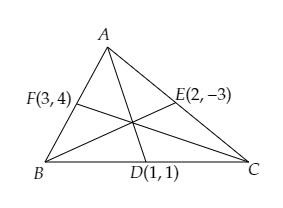# If the coordinates of the mid-points of the sides of a triangle are $(1, 1), (2, -3)$ and $(3, 4)$, find the vertices of the triangle.

Given:

The coordinates of the mid-points of the sides of a triangle are $(1, 1), (2, -3)$ and $(3, 4)$.

To do:

We have to find the vertices of the triangle.

Solution:

Let $A (x_1, y_1), B (x_2, y_2)$ and $C (x_3, y_3)$ be the vertices of the $\triangle ABC$ and $D(1,1), E(2,-3)$ and $F(3,4)$ are the mid-points of $BC, CA$ and $AB$.$D$ is the mid-point of $BC$.

This implies,

$\frac{x_{2}+x_{3}}{2}=1$

$\Rightarrow x_{2}+x_{3}=2$.....(i)
$\frac{y_{2}+y_{3}}{2}=1$

$\Rightarrow y_{2}+y_{3}=2$......(a)
Similarly,

$E$ is the mid-point of $A C$.
$\frac{x_{3}+x_{1}}{2}=2$

$\Rightarrow x_{3}+x_{1}=4$.......(ii)

$\frac{y_{3}+y_{1}}{2}=-3$

$\Rightarrow y_{3}+y_{1}=-6$.......(b)
$\mathrm{F}$ is the mid-point of $\mathrm{AB}$.
$\frac{x_{2}+x_{1}}{2}=3$

$\Rightarrow x_{2}+x_{1}=6$........(iii)

$\frac{y_{2}+y_{1}}{2}=4$

$\Rightarrow y_{2}+y_{1}=8$.......(c)

Adding (i), (ii) and (iii), we get,

$2\left(x_{1}+x_{2}+x_{3}\right)=12$

$\Rightarrow x_{1}+x_{2}+x_{3}=6$......(iv)

Subtracting (i), (ii) and (iii) from (iv), we get,

$x_{1}=4, x_{2}=2, x_{3}=0$
Similarly,
Adding (a), (b) and (c), we get,

$2\left(y_{1}+y_{2}+y_{3}\right)=4$

$\Rightarrow y_{1}+y_{2}+y_{3}=2$.......(d)
Subtracting (a),(b) \) and (c) from (d), we get,

$y_{1}=0$
$y_{2}=8$
$y_{3}=-6$
Therefore, vertices of $\Delta \mathrm{ABC}$ are $\mathrm{A}(4,0), \mathrm{B}(2,8), \mathrm{C}(0,-6)$

The vertices of the triangle are $(4,0), (2,8)$ and $(0,-6)$.

Updated on: 10-Oct-2022

23 Views